Chapter 4: Rigid Bodies

# 4.5 Examples

Here are examples from Chapter 4 to help you understand these concepts better. These were taken from the real world and supplied by FSDE students in Summer 2021. If you’d like to submit your own examples, please send them to the author eosgood@upei.ca.

# Example 4.5.1: External Forces, submitted by Elliott Fraser

1. Problem

Billy (160 lbs), Bobby (180 lbs), and Joe (145 lbs) are walking across a small bridge with a length of 11 feet. Both sides of the bridge are supported by rollers. Billy is 2 feet along the bridge whereas Joe is 9 feet along the bridge. If the maximum force that the left side of the bridge can withstand without failing is 225 lbs, where along the bridge can Bobby stand?

Real-life scenario:

2.Draw

Sketch: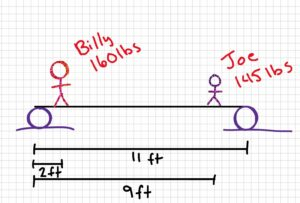Free-body diagram: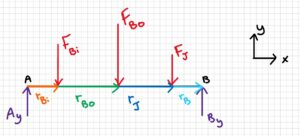3. Knowns and Unknowns

Known:

• rBi = 2 ft
• rJ = 9 ft
• rB = 11 ft
• FBi = 160 lb
• FJ = 145 lb
• FBo = 180 lb
• Ay = 225 lb (since this is the maximum force without failure)

Note: Since the mass of the bridge was not given, we assume it is negligible and ignore it for this question.

Unknown: rBo

4. Approach

Use equilibrium equations ( $\sum\underline{F}=0$ , $\sum\underline{M}=0$ . Use sum of forces in y to find By; use sum of moments to find where Bobby can stand. Solve for x.

5. Analysis

$$\sum F_y=0=-F_{Bi}-F_{Bo}-F_J+A_y+B_y\\\\B_y=F_{Bi}+F_{Bo}+F_J-A_y\\\\B_y=160 lb+180 lb+145 lb-225 lb\\\\\\B_y=260 lb$$

$$\sum M_A=0=-(F_{Bi})(r_{Bi})-(F_{Bo})(r_{Bo})-(F_{J})(r_{J})+(B_{y})(r_{B})\\r_{bo}=\frac{-(F_{Bi})(r_{Bi})-(F_{J})(r_{J})+(B_{y})(r_{B})}{F_{Bo}}\\r_{Bo}=\frac{-(160 lb)(2 ft)-(145 lb)(9 ft)+(260 lb)(11 ft)}{180 lb}$$

$$\underline{r_{Bo}=6.86 ft}$$

6. Review

Bobby can stand anywhere from 6.8611 ft – 11 ft from A with no problems. If Bobby were to stand between 0 ft and 6.811 ft, the left side of the bridge would fail.

# Example 4.5.2: Free-Body Diagrams, submitted by Victoria Keefe

1. Problem

A box is sitting on an inclined plane (θ = 15°) and is being pushed down the plane with a force of 20 N. Draw the free body diagram for the box, while it is in static equilibrium.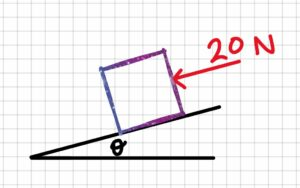2. Draw3. Knowns and Unknowns

θ = 15°

FA

Unknowns: free-body diagram of box

4. Approach

Draw the box, then draw all forces acting on it

5. Analysis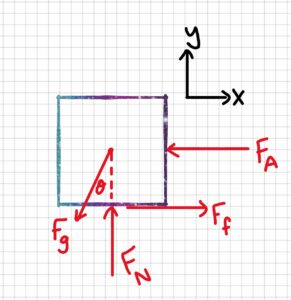6. Review

All forces acting upon the box are drawn, including weight/gravitational force, normal force, friction, and applied forces.

# Example 4.5.3: Friction, submitted by Deanna Malone

1. Problem

A box is being pushed along level ground with a force of 150 N at an angle of 30 with the horizontal. The mass of the box is 12 kg.

a) What is the normal force between the box and the floor?

b) What is the coefficient of friction between the box and the floor?

2. Draw

Sketch: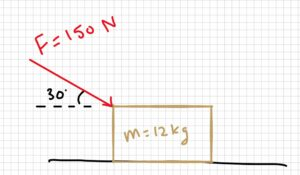Free Body Diagram: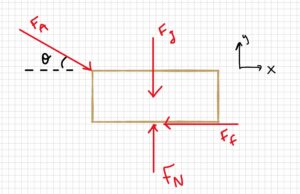3. Knowns and Unknowns

Knowns:

• FA = 150 N
• θ = 30°
• m = 12 kg

Unknowns: FN, μ

4. Approach

Use equilibrium equations ( $\sum\underline{F}=0$ , $\sum\underline{M}=0$ ), SOH CAH TOA, friction equation

5. Analysis

Part a:

Find Fg:

$$Fg=m\cdot g\\Fg=(12kg)(9.81m/s^2)\\\\Fg=117.72N$$

Find FN using equilibrium equations:

$$\sum Fy=0=F_N-F_g-F_A\sin 30^{\circ}\\0=F_N-117.72N-150N\cdot \sin 30^{\circ}\\F_N=117.72+150N\cdot\sin 30^{\circ}\\\\\underline{F_N=192.7 N}$$

Part b:

Find two equations for Ff, set equal, solve for μ

$$\sum F_x=0=F_A\cos 30^{\circ} -F_f\\0=150N\cdot\cos 30^{\circ}-F_f$$

$$F_f=150N\cdot\cos 30^{\circ}\\F_f=M\cdot F_N\\150N\cdot\cos 30^{\circ}=\mu\cdot F_N$$

$$\mu=\frac{150N\cdot\cos 30^{\circ}}{F_N}\\\mu=\frac{150N\cdot\cos 30^{\circ}}{192.72N}=0.67405$$

$$\underline{\mu=0.67}$$

6. Review

FN, Fg, and the y component of FA are the only forces in the y direction so it makes sense that they need to equal zero for the equilibrium equations. FN is the only positive force in the y direction, so it makes sense that it equals the magnitude of the other two put together.

The coefficient found between the box and the floor is reasonable as it is less than 1, and it’s reasonable for a box on the floor. For example, if the box was wood and the floor was wood, the coefficient of static friction would be anywhere from 0.5-0.7, so having a coefficient of friction being equal to 0.67 makes sense.

# Example 4.5.4: Friction, submitted by Dhruvil Kanani

1. Problem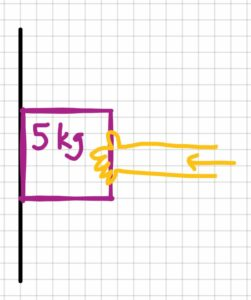A person is trying to prevent a brick from sliding on a rough vertical surface by applying force in the direction of wall. Assuming the coefficient of static friction is 0.49 and mass of the brick is 5 kg,

• a) Determine the minimum force required to prevent the brick from slipping.
• b) Find the distributed load or intensity if the length of the person’s hands from the tip of his fingers to their wrist is 16 cm.

2. Draw

Sketch:

Free-body diagram (box):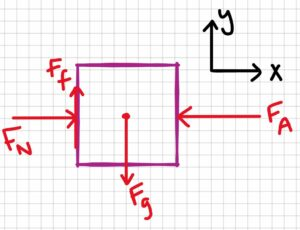Free-body diagram (distributed load):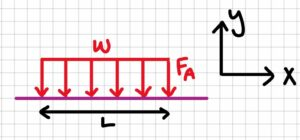3. Knowns and Unknowns

Knowns:

• Mass of brick (m) = 5kg
• Coefficient of friction (μ1) = 0.49
• Acceleration due to gravity (g) = 9.8m/s2
• Length of the hand (L) = 16 cm

Unknowns:

Applied force (FA), intensity (w)

4. Approach

Use equilibrium equations ( $\sum\underline{F}=0$ , $\sum\underline{M}=0$ ), equations for Fg and Ff (see below).

$$F_g=m g\\F_f=\mu F_N$$

5. Analysis

Part a:

$$F_g=m\cdot g\\F_g=5kg\cdot 9.81m/s^2\\F_g=49.05N$$

$$\sum F_y=0=-F_g+F_f\\F_f=F_g\\F_f=49.05N$$

$$F_f=\mu_1 F_N\\F_N=\frac{F_f}{\mu_1}\\F_N=\frac{49.05N}{0.49}$$

$$\underline{F_N=100.1N}$$

Part b:

$$\sum F_x=0=F_N-F_A\\F_A=F_N\\F_A=100.1N$$

$$w=\frac{F}{L}\\w={100.1N}{(16cm\times\frac{1m}{100cm})}$$

$$\underline{w=625N/m}$$

6. Review

It makes sense that the applied force is larger than the gravitational force. It also makes sense that the normal and applied forces are equal, since they are the only forces in the x direction (same goes for the friction and gravitational forces).

# Example 4.5.5: Friction, submitted by Emma Christensen

1. Problem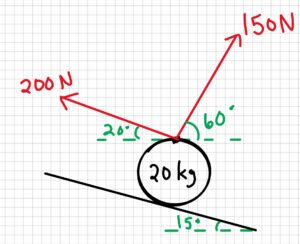A ball is suspended by two ropes, and rests on an inclined surface with angle 15°. Rope A pulls on the ball with force 200 N, and rope B has force 150 N. They each have angles of 20° and 60° from the inclined surface plane, as shown in the image below.

a) Draw a free-body diagram of the ball

b) Find the friction force

2. Draw

Sketch: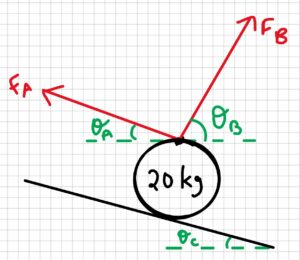3. Knowns and Unknowns

• θA = 20°
• θB = 60°
• θC = 15°
• m = 20 kg
• FA = 200 N
• FB = 150 N

Unknowns: Ff

4. Approach

Draw the ball, then add forces. Use equilibrium equations ( $\sum\underline{F}=0$ , $\sum\underline{M}=0$ ) to find the friction force.

5. Analysis

Part a: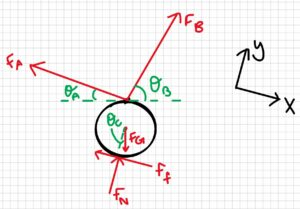Part b:

Step 1: find FG

$$F_G=m g\\F_G=20kg\cdot 9.81m/s^2\\F_G=196.2N$$

Step 2: Find the x-component of FG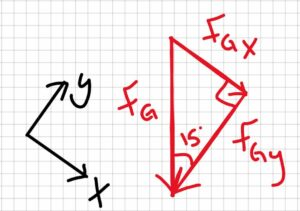$$F_{GX}=F_G\sin(15^{\circ})\\F_{GX}=196.2N\cdot\sin(15^{\circ})\\F_{GX}=50.78N$$

Step 3: Find the x-component of FA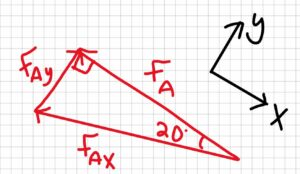$$\cos(20^{\circ})=\frac{F_A}{F_{AX}}\\F_{AX}=\frac{F_A}{\cos(20^{\circ})}\\F_{AX}=\frac{200N}{\cos(20^{\circ})}\\F_{AX}=212.8N$$

Step 4: Find the x-component of FB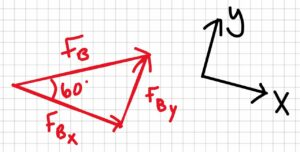$$F_{BX}=F_B\cos(60^{\circ})\\F_{BX}=150N\cos(60^{\circ})\\F_{BX}=75N$$

Step 5: Sum forces in the x-direction to find the frictional force

$$\sum F_x=0=-F_f+f_{BX}-F_{AX}+F_{GX}\\F_f=-F_{AX}+F_{BX}+F_{GX}\\F_f=-212.8N+75N-50.78N$$

$$\underline{F_f=-87.02N}$$

Because the frictional force is negative, that means the frictional force actually acts in the opposite direction, so the friction is keeping the ball from going up the plane.

6. Review

The units of Ff are newtons, which makes sense because it is a force. It also makes sense that FAx is larger than FGx and FBx.